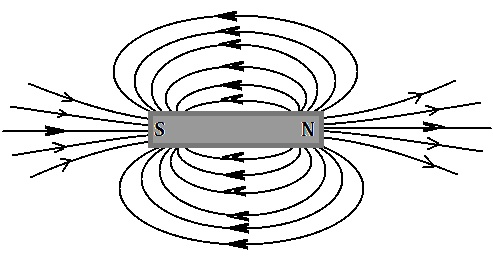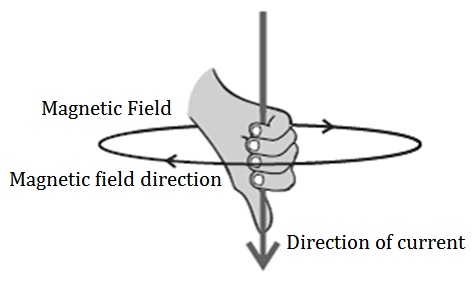Study Materials:
CBSE NOTES ⇒ Class 10th ⇒ Science ⇒ 13. Magnetic Effects of Electric Current

## 13. Magnetic Effects of Electric Current

### Introduction

Chapter 13. Magnetic Effects of Electric Current​

Magnetic field: The region surrounding a magnet, in which the force of the magnet can be detected, is called a magnetic field.

Magnetic field lines: There are many lines forms around a magnet, which are originated from north pole and it seems to end with south pole of magent such line are known as magnetic field lines.Properties of magnetic field lines:

(i) The magentic field lines emerge from north pole and merge at the south pole.

(ii) Inside the magnet, the direction of field lines is from its south pole to its north pole.

(iii) The magnetic field lines are closed curves.

(iv) The magnetic field is stronger, where the field lines are crowded.

(v) Two field lines do not intersect/cross each other.

Magnetic field lines do not intersect each other:

This is so, magnetic field lines do not intersect each other. If they did, it would
mean that at the point of intersection, the compass needle would point
towards two directions, which is not possible.

Magnetic field around a current-carrying conductor:

• An electric current through a metallic conductor produces a magnetic field around it.
• When a current carrying conductor is kept over a compass and parallel to its needle, when the direction of flowing of electric current reversed the deviation of compass is in opposite direction.
• If the current is increased, the deflection also increases.
• The magnitude of the magnetic field produced at a given point increases as the current through the wire increases.
• When we place the compass at a farther point from the conducting
wire, the deflection in the needle decreases.

Right-Hand Thumb Rule:

Imagine that you are holding a current-carrying straight conductor in your right hand such that the thumb points towards the direction of current. Then your fingers will wrap around the conductor in the direction of the field lines of the magnetic field. This is known as the right-hand thumb rule. This rule is also known as Maxwell’s corkscrew ruleMagnetic Field due to a Current through a Circular Loop:

The magnetic field produced by a current-carrying straight wire depends inversely on the distance from it. Similarly at every point of a current-carrying circular loop, the concentric circles representing the magnetic field around it would become larger and larger as we move away from the wire.

Properties of magnetic field line of a current through a circular loop:

(i) At the center of this loop the magnetic field lines are a straight line.

(ii) Every point on the wire carrying current would give rise to the magnetic field appearing as straight lines at the center of the loop.

(iii) Section of the wire contributes to the magnetic field lines in the same direction within the loop.• The magnetic field produced by a current-carrying wire at a given point depends directly on the current passing through it.
• if there is a circular coil having n turns, the field produced is n times as large as that produced by a single turn. This is because the current in each circular turn has the same direction, and the field due to each turn then just adds up.

Other Pages of this Chapter:

Important Study materials for classes 06, 07, 08,09,10, 11 and 12. Like CBSE Notes, Notes for Science, Notes for maths, Notes for Social Science, Notes for Accountancy, Notes for Economics, Notes for political Science, Noes for History, Notes For Bussiness Study, Physical Educations, Sample Papers, Test Papers, Mock Test Papers, Support Materials and Books.Mathematics Class - 11th

NCERT Maths book for CBSE Students.

books

## Study Materials List:

##### CBSE NOTES ⇒ Class 10th ⇒ Science
1. Chemical Reactions and Equations
2. Acids, Bases and Salts
3. Metals and Non-metals
4. Carbon and its Compounds
5. Periodic Classification of Elements
6. Life Processes
7. Control and Coordination
8. How do Organisms Reproduce
9. Heredity and Evolution
10. Light-Reflection and Refraction
11. Human Eye and Colourful World
12. Electricity
13. Magnetic Effects of Electric Current
14. Sources of Energy
15. Our Environment
16. Management of Natural Resources

New Books

See More ...